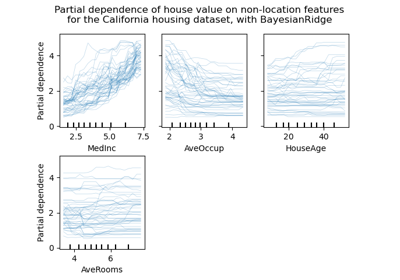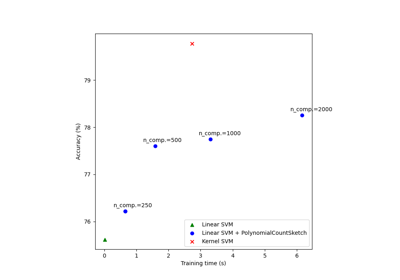# sklearn.kernel_approximation.PolynomialCountSketch¶

class sklearn.kernel_approximation.PolynomialCountSketch(*, gamma=1.0, degree=2, coef0=0, n_components=100, random_state=None)[source]

Polynomial kernel approximation via Tensor Sketch.

Implements Tensor Sketch, which approximates the feature map of the polynomial kernel:

K(X, Y) = (gamma * <X, Y> + coef0)^degree


by efficiently computing a Count Sketch of the outer product of a vector with itself using Fast Fourier Transforms (FFT). Read more in the User Guide.

New in version 0.24.

Parameters:
gammafloat, default=1.0

Parameter of the polynomial kernel whose feature map will be approximated.

degreeint, default=2

Degree of the polynomial kernel whose feature map will be approximated.

coef0int, default=0

Constant term of the polynomial kernel whose feature map will be approximated.

n_componentsint, default=100

Dimensionality of the output feature space. Usually, n_components should be greater than the number of features in input samples in order to achieve good performance. The optimal score / run time balance is typically achieved around n_components = 10 * n_features, but this depends on the specific dataset being used.

random_stateint, RandomState instance, default=None

Determines random number generation for indexHash and bitHash initialization. Pass an int for reproducible results across multiple function calls. See Glossary.

Attributes:
indexHash_ndarray of shape (degree, n_features), dtype=int64

Array of indexes in range [0, n_components) used to represent the 2-wise independent hash functions for Count Sketch computation.

bitHash_ndarray of shape (degree, n_features), dtype=float32

Array with random entries in {+1, -1}, used to represent the 2-wise independent hash functions for Count Sketch computation.

n_features_in_int

Number of features seen during fit.

New in version 0.24.

feature_names_in_ndarray of shape (n_features_in_,)

Names of features seen during fit. Defined only when X has feature names that are all strings.

New in version 1.0.

AdditiveChi2Sampler

Approximate feature map for additive chi2 kernel.

Nystroem

Approximate a kernel map using a subset of the training data.

RBFSampler

Approximate a RBF kernel feature map using random Fourier features.

SkewedChi2Sampler

Approximate feature map for “skewed chi-squared” kernel.

sklearn.metrics.pairwise.kernel_metrics

List of built-in kernels.

Examples

>>> from sklearn.kernel_approximation import PolynomialCountSketch
>>> from sklearn.linear_model import SGDClassifier
>>> X = [[0, 0], [1, 1], [1, 0], [0, 1]]
>>> y = [0, 0, 1, 1]
>>> ps = PolynomialCountSketch(degree=3, random_state=1)
>>> X_features = ps.fit_transform(X)
>>> clf = SGDClassifier(max_iter=10, tol=1e-3)
>>> clf.fit(X_features, y)
SGDClassifier(max_iter=10)
>>> clf.score(X_features, y)
1.0


Methods

 fit(X[, y]) Fit the model with X. fit_transform(X[, y]) Fit to data, then transform it. get_feature_names_out([input_features]) Get output feature names for transformation. get_params([deep]) Get parameters for this estimator. set_output(*[, transform]) Set output container. set_params(**params) Set the parameters of this estimator. Generate the feature map approximation for X.
fit(X, y=None)[source]

Fit the model with X.

Initializes the internal variables. The method needs no information about the distribution of data, so we only care about n_features in X.

Parameters:
X{array-like, sparse matrix} of shape (n_samples, n_features)

Training data, where n_samples is the number of samples and n_features is the number of features.

yarray-like of shape (n_samples,) or (n_samples, n_outputs), default=None

Target values (None for unsupervised transformations).

Returns:
selfobject

Returns the instance itself.

fit_transform(X, y=None, **fit_params)[source]

Fit to data, then transform it.

Fits transformer to X and y with optional parameters fit_params and returns a transformed version of X.

Parameters:
Xarray-like of shape (n_samples, n_features)

Input samples.

yarray-like of shape (n_samples,) or (n_samples, n_outputs), default=None

Target values (None for unsupervised transformations).

**fit_paramsdict

Returns:
X_newndarray array of shape (n_samples, n_features_new)

Transformed array.

get_feature_names_out(input_features=None)[source]

Get output feature names for transformation.

The feature names out will prefixed by the lowercased class name. For example, if the transformer outputs 3 features, then the feature names out are: ["class_name0", "class_name1", "class_name2"].

Parameters:
input_featuresarray-like of str or None, default=None

Only used to validate feature names with the names seen in fit.

Returns:
feature_names_outndarray of str objects

Transformed feature names.

get_params(deep=True)[source]

Get parameters for this estimator.

Parameters:
deepbool, default=True

If True, will return the parameters for this estimator and contained subobjects that are estimators.

Returns:
paramsdict

Parameter names mapped to their values.

set_output(*, transform=None)[source]

Set output container.

See Introducing the set_output API for an example on how to use the API.

Parameters:
transform{“default”, “pandas”}, default=None

Configure output of transform and fit_transform.

• "default": Default output format of a transformer

• "pandas": DataFrame output

• None: Transform configuration is unchanged

Returns:
selfestimator instance

Estimator instance.

set_params(**params)[source]

Set the parameters of this estimator.

The method works on simple estimators as well as on nested objects (such as Pipeline). The latter have parameters of the form <component>__<parameter> so that it’s possible to update each component of a nested object.

Parameters:
**paramsdict

Estimator parameters.

Returns:
selfestimator instance

Estimator instance.

transform(X)[source]

Generate the feature map approximation for X.

Parameters:
X{array-like}, shape (n_samples, n_features)

New data, where n_samples is the number of samples and n_features is the number of features.

Returns:
X_newarray-like, shape (n_samples, n_components)

Returns the instance itself.

## Examples using sklearn.kernel_approximation.PolynomialCountSketch¶Release Highlights for scikit-learn 0.24

Release Highlights for scikit-learn 0.24Scalable learning with polynomial kernel approximation

Scalable learning with polynomial kernel approximation